Related Articles
Underscore.js | each() function
• Last Updated : 14 Feb, 2019

Underscore.js is a JavaScript library that provides a lot of useful functions that helps in the programming in a big way like the map, filter, invoke etc even without using any built-in objects.

The _.each() function is an inbuilt function in Underscore.js library of JavaScript which is used to return the each element of the given list.

Syntax:

`_.each(list, function)`

Parameters: It accepts two parameters which are specified below:

• list: It is the list which contains some elements.
• function: It is the function which is executed by taking each element of the list.

Return values: It returns each element of the list.

JavaScript code to show the working of this function:

• Passing a list of numbers with alert() function: When the list of elements is passed to the alert inbuilt function then it takes the element from the list one by one and then shows it on the page (which is the function of the alert function). After alerting all the elements, the alert function ends and the _.each function also ends.

 ` ` ` `  ` ` `    `` ` `    `` ` ` `  `    `` ` ` ` ` `  ` ` `    `` ` ` ` ` `  ` `

Output: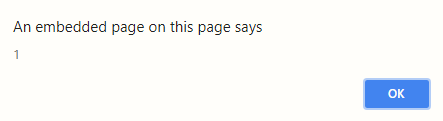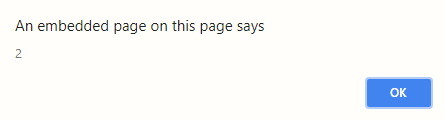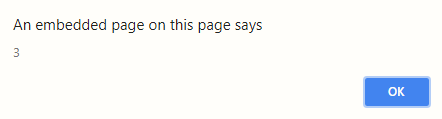• Passing a list of words with prompt() function: When the list of words is passed to the prompt inbuilt function then it takes the words from the list one by one and then shows it on the page along with its index fron the list (which is the function of the prompt function). After alerting all the elements, the prompt function ends and the _.each function also ends.

 ` ` ` `  ` ` `    `` ` `    `` ` ` `  `    `` ` ` ` ` `  ` ` `    `` ` ` ` ` `  ` `

Output: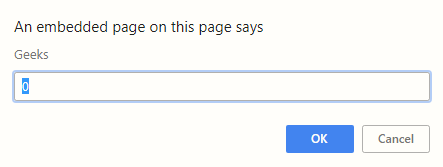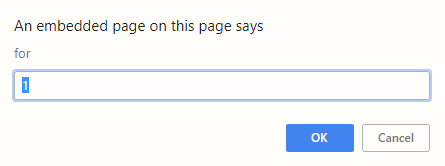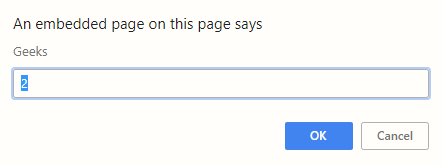• Passing a list of numbers with a user defined function: First we need to create a function by using the ‘function’ keyword. Then, when we pass the list of elements to the user defined function then it takes the element from the list one by one and print it. After all the elements are passed, the function ends. And the _.each function also ends.

 ` ` ` `  ` ` `    `` ` `    `` ` ` `  `    `` ` ` ` ` `  ` ` `    `` ` ` ` ` `  ` `

Output:

```Hi Geeks
Hi Geeks
Hi Geeks```My Personal Notes arrow_drop_up
Recommended Articles
Page :